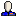After working on the Wikipedia Antimatroid article this weekend, I was excited to realize that my paper on maximum subtrees withchouyu_31 from SWAT'06 could be interpreted as an antimatroid algorithm: there is an antimatroid whose convex sets are subtrees of a tree, so this problem is finding maximum weight convex sets in certain kinds of antimatroids. Could it be generalized to all antimatroids?

Given a graph (on which we wish to find a vertex cover), construct a path poset with sets $$\{ v \}$$ for each vertex in $$G$$ and $$\{ u, \{ u,v \} \}$$ and $$\{ v, \{ u,v \} \}$$ for each edge $$\{ u,v \}$$ in G. Weight each vertex $$+1$$ and each edge $$-n$$. Then a convex set in the antimatroid represented by this path poset is formed by removing some vertices from $$G$$ and some edges covered by the removed vertices. The maximum weight convex set has all edges removed (because their weights are too negative) and only as few vertices removed as needed to cover all edges. Its weight is $$n-C$$ where $$C$$ is the size of the optimal vertex cover.